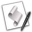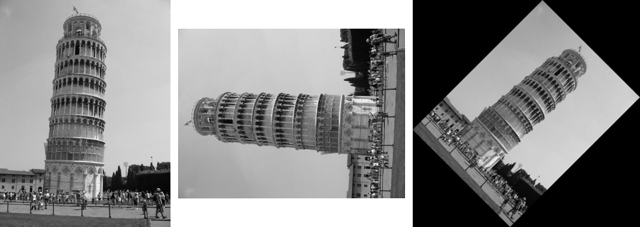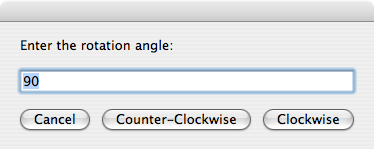## Rotating an Image

From the Image Events dictionary:

rotate v : Rotate an image
rotate (reference): the object for the command
to angle (real): rotate using an angle

The rotate command is used to rotate an image clockwise around its center point. The value for the to angle parameter is a positive integer from 1 to 359.

To convert a negative rotation angle (counter-clockwise values), such as -90, to a positive value, add 360:

-90 + 360 = 270

NOTE: Images rotated to values other than 90, 180, or 270 will have their “non-image” areas padded with black pixels to maintain the resulting image shape as a rectangle.

To rotate an image, follow the same steps as the script used to flip an image.set this_file to choose file
try
tell application "Image Events"
-- start the Image Events application
launch
-- open the image file
set this_image to open this_file
-- perform action
rotate this_image to angle 270
-- save the changes
save this_image with icon
-- purge the open image data
close this_image
end tell
on error error_message
display dialog error_message
end tryUse of the roate command. (L to R) Normal, rotated 270 degrees or -90 degrees (90 degress counter-clockwise), rotated 45 degrees (note the automatic padding of the space around the rotated image).

### Example Accepting User Inputwill allow the user to enter the rotation information when the script is executed:set this_file to choose file without invisibles
set the rotation_angle to 90
repeat
display dialog ¬
"Enter the rotation angle:" default answer rotation_angle ¬
buttons {"Cancel", "Counter-Clockwise", "Clockwise"}
copy the result as list to {rotation_angle, rotation_direction}
try
set rotation_angle to rotation_angle as number
if the rotation_angle is greater than 0 and ¬
the rotation_angle is less than 360 then
if the rotation_direction is "Counter-Clockwise" then
set the rotation_angle to 360 - the rotation_angle
end if
exit repeat
end if
on error
beep
end try
end repeat
try
tell application "Image Events"
-- start the Image Events application
launch
-- open the image file
set this_image to open this_file
-- perform action
rotate this_image to angle rotation_angle
-- save the changes
save this_image with icon
-- purge the open image data
close this_image
end tell
on error error_message
display dialog error_message
end try